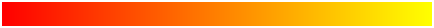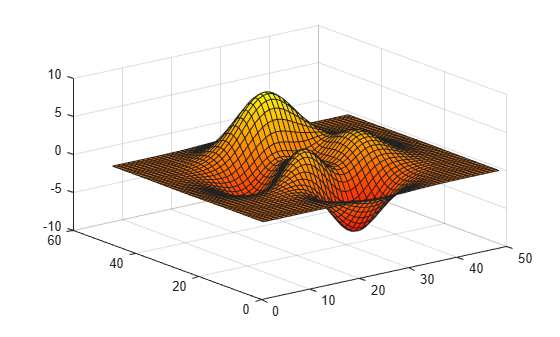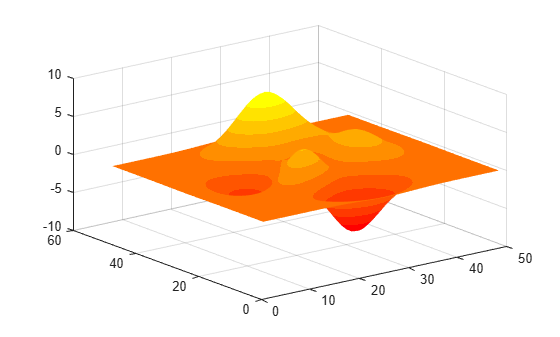# autumn

Autumn colormap array

## Syntax

``c = autumn``
``c = autumn(m)``

## Description

example

````c = autumn` returns the autumn colormap as a three-column array with the same number of rows as the colormap for the current figure. If no figure exists, then the number of rows is equal to the default length of 256. Each row in the array contains the red, green, and blue intensities for a specific color. The intensities are in the range [0,1], and the color scheme looks like this image.```

example

````c = autumn(m)` returns the colormap with `m` colors.```

## Examples

collapse all

Plot a surface and assign the autumn colormap.

```surf(peaks); colormap('autumn');```Get the autumn colormap array and reverse the order. Then apply the modified colormap to the surface.

```c = autumn; c = flipud(c); colormap(c);```Get a downsampled version of the autumn colormap containing only ten colors. Then display the contours of the peaks function by applying the colormap and interpolated shading.

```c = autumn(10); surf(peaks); colormap(c); shading interp;```## Input Arguments

collapse all

Number of colors, specified as a scalar integer value. The default value of `m` is equal to the length of the colormap for the current figure. If no figure exists, the default value is 256.

Data Types: `single` | `double`

## Version History

Introduced before R2006a

expand all